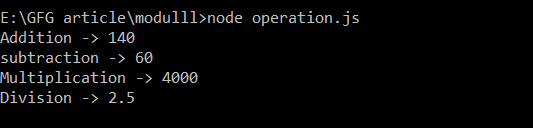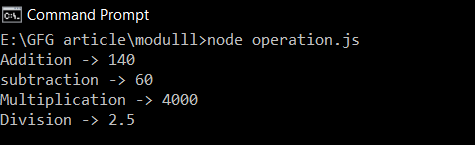# Difference between module.exports and exports in Node.js

• Last Updated : 07 Oct, 2021

The module is a plain JavaScript Object representing the current module. It is local to each module and also it is private. It has exports property which is a plain JavaScript variable, set to module.exports. At the end of the file, Node.js return module.exports to the required function.

When we want to export a single class/variable/function from one module to another module, we use module.exports way.

Example: Create two file calculator.js and operation.js and export the Arithmetic class from calculator.js to operation.js using module.exports method. Here, we have created a class Arithmetic and exported the whole class using module.exports.

Filename: calculator.js

## Javascript

 `class Artimatics {``    ``constructor(a, b) {``        ``this``.a = a;``        ``this``.b = b;``    ``}`` ` `    ``add() {``        ``return` `this``.a + ``this``.b;``    ``}``    ``subtract() {``        ``return` `this``.a - ``this``.b;``    ``}`` ` `    ``multiply() {``        ``return` `this``.a * ``this``.b;``    ``}`` ` `    ``divide() {``        ``if` `(``this``.b != 0) {``            ``return` `this``.a / ``this``.b;``        ``}``        ``return` `"divided by zero !!!!"``;``    ``}``};`` ` `module.exports = Artimatics;`

Filename: operation.js

## Javascript

 `const Artimatics = require(``'./calculator.js'``);`` ` `const op = ``new` `Artimatics(100,40);`` ` `console.log(`Addition -> \${op.add()}`);``console.log(`subtraction -> \${op.subtract()}`);``console.log(`Multiplication -> \${op.multiply()}`);``console.log(`Division -> \${op.divide()}`);`

Run the operation.js file using the following command:

`node operation.js`

Output:Using module.exports

When we want to export multiple variables/functions from one module to another, we use exports.

Example: Create a two file calculator.js and operation.js and export multiple functions from calculator.js file.

Filename: calculator.js

## Javascript

 `exports.add = (a, b) => a + b;``exports.subtract = (a, b) => a - b;``exports.multiply = (a, b) => a * b;``exports.divide = (a, b) => {``    ``if` `(b != 0) {``        ``return` `a / b;``    ``}``    ``return` ``Divided by zero !!!`;``}`

Filename: operation.js

## Javascript

 `const Artimatics = require(``'./calculator.js'``);`` ` `console.log(`Addition -> \${Artimatics.add(100,40)}`);``console.log(`subtraction -> \${Artimatics.subtract(100,40)}`);``console.log(`Multiplication -> \${Artimatics.multiply(100,40)}`);``console.log(`Division -> \${Artimatics.divide(100,40)}`);`

Run the operation.js file using the following command:

`node operation.js`

Output:Using exports

Key difference between module.exports and exports:

My Personal Notes arrow_drop_up